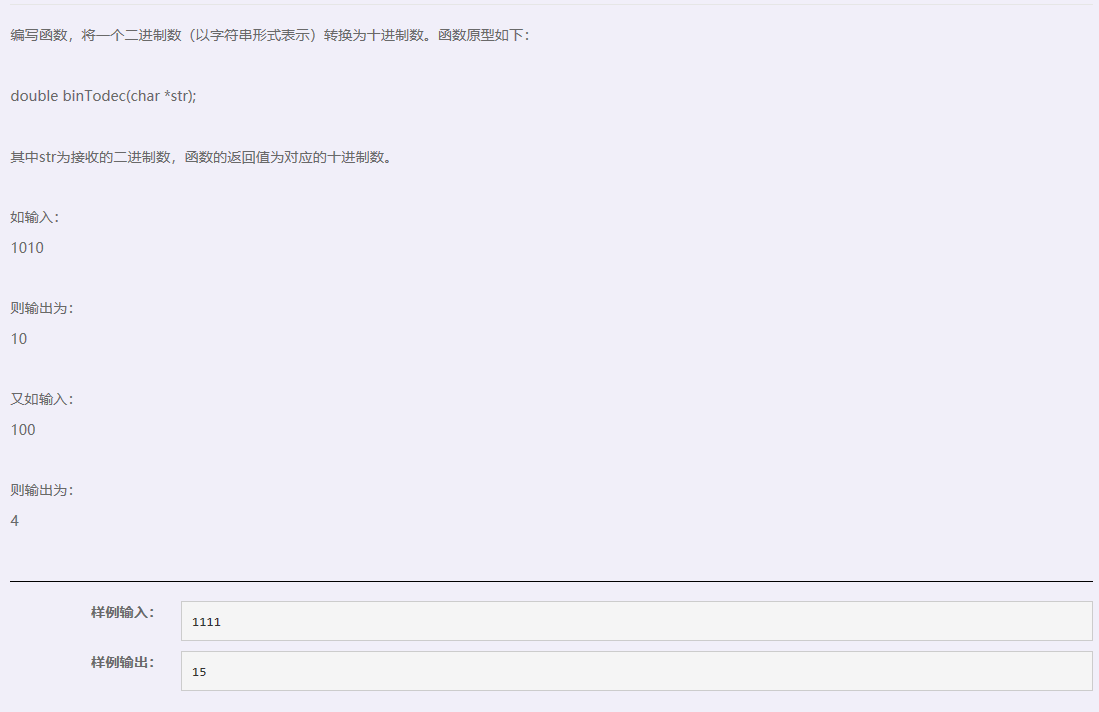• c语言编程-二进制转化为十进制 #include<stdio.h> static int a=0; double bintodec(char*str) { a=a*2+(*str-'0'); return 0; } int main() { char ch; while(ch=getchar(),ch!='\n') { char *p=&...
c语言编程-二进制转化为十进制#include<stdio.h>
static int a=0;
double bintodec(char*str)
{
a=a*2+(*str-'0');
return 0;
}
int main()
{
char ch;
while(ch=getchar(),ch!='\n')
{
char *p=&ch;
bintodec(p);
}
printf("%d",a);
}
如果不要求调用函数，可以直接在主函数中实现如下指令：
#include<stdio.h>
int main()
{
char ch;
int a=0;
while(ch=getchar(),ch!='\n')
a=a*2+(ch-'0');
printf("%d\n",a);
return 0;
}


展开全文• 十进制转化为二进制并输出（以整型为例） 首先需要明确十进制转为二进制的算法：将所需转化的数除以2，所得余数为k1，将所得商再除以2，所得余数为k2…...重复步骤直到商为0；读数时从最后一个余数读起，即kn,k(n-1)...
十进制转化为二进制并输出（以整型为例）
首先需要明确十进制转为二进制的算法：将所需转化的数除以2，所得余数为k1，将所得商再除以2，所得余数为k2…...重复步骤直到商为0；读数时从最后一个余数读起，即kn,k(n-1)…k2,k1；所得数便是转化成的二进制数（除二取余法）
例如：将20转化为二进制数
20/2   商为10   余数为0   k1
10/2   商为5     余数为0   k2
5/2     商为2     余数为1   k3
2/2     商为1     余数为0   k4
1/2     商为0     余数为1   k5
故二进制数为：10100

编程思路：定义输入的函数为input，商为quotient，余数为remainder；则可以用一个数组result来存放每次计算后所得的余数，然后倒序输出

代码如下：

#include <stdio.h>
int main(void) {

//提示用户输入数据
int input = 0 ;
printf("请输入一个数据：");
scanf("%d", &input);

//十进制转为二进制
int quotient = input;
int remainder = 0;
int result ;
int  i = 0;

while (quotient != 0) {
remainder = quotient % 2;      //求余数
result [i] = remainder;       //数组存放每次所得的余数
i++;
quotient = quotient / 2;     //求商
}
i--;      //while循环中多加了一次i，需要减去

//倒序输出数组
printf("转化成二进制为：");
for ( ; i >= 0 ; --i) {
printf("%d", result[i]);
}
printf("\n");
return 0 ;
}

但是在这种情况中，由于不知道输入数据的大小，存在事先定义好的数组存放不下所有余数的情况。如果将所有余数化为一个具体的值，则更具有适应性，也方便输出

思路：定义输出的值为result， 可以发现result的个位等于k1，十位等于k2......定义time来记录result进位的次数，每进一次即time*10；则可以通过time*k来控制result的个十百千万....位

代码如下：

#include <stdio.h>
int main(void) {

//提示用户输入数据
int input;

printf("请输入一个数据：");
scanf("%d", &input);

//将数字转成二进制
int quotient = input;
int remainder = 0;
int result = 0;
int time = 1;

while (quotient != 0 ) {
remainder = quotient % 2;       //求余数
result += remainder * time;    //将余数化为一个具体的值
quotient = quotient / 2;      //求商
time *= 10;
}

//输出二进制数据
printf("转化成二进制为：%d\n",result);

return 0;
}


展开全文• 最近在朋友解决ip地址比较的时候，在二进制转化卡了好久，因为最后的结果保存在数组中，难以操作。 在网上找了好久的方法，也没有能令自己满意的，于是便一鼓作气，硬着头皮敲出了一种新的转变方式。 （ps：因为在...
一种全新的转变方法，不再使用数组来保存数据。
最近在为朋友解决ip地址比较的时候，在二进制转化卡了好久，因为最后的结果保存在数组中，难以操作。
在网上找了好久的方法，也没有能令自己满意的，于是便一鼓作气，硬着头皮敲出了一种新的转变方式。
（ps：因为在网上没有发现这种方法，如有先者早已发布，多有冒犯，见谅见谅，虽然代码很短，但也是一种创新）
新的改变
在网上的所有方法中，都是利用了for循环来对每个数进行余2法进行转化，然后挨个的放到数组中，再利用for循环一个个输出，这就导致结果只是一个由0和1组成的字符串，难以进行其他操作，本代码，将结果直接转变为一个数字，可以直接操作。
代码运行示例
图片:代码思路
从正常的数学思路求解二进制的方法可得，所除得到的第一个数在二进制中为最后一位，第二个为第二位，所以只要将数字放到相应的位上即可。放到位上，各个位相差10，所以只要让余下的0和1乘以相应的10的次幂即可。
完整源码
//头文件
#include<stdio.h>
#include<math.h>
//主函数
int main()
{
int ip;
int i=1,j,k,l,sum;
printf("请输入一个整数：");
scanf("%d",&ip);
//i控制循环
while(ip > 0 && i <= 8)
{
//j为余数，k保存j，因为在循环中，j如若为1，会因为在第二个for循环中乘到0而变成0，所以提前保存一下。
j=ip%2;
k=j;
ip=ip/2;
for(l=i-1;l<i;l++)
{
j=j*pow(10,l);
sum+=j;
//每次循环后，都将j的值还原
j=k;
}
i++;
}
printf("该数的二进制为（不满八位则自动补齐为0）：%08d",sum);
return 0;
}

注
这是我第一次编写博客，如有不足希望指出，如有冒犯希望包含，如若侵权，希望你能在评论和私信中告知，我会道歉并删除哦


展开全文• c语言二进制转化为十进制Here you will get ... 在这里，您将获得将C中的十进制转换为二进制的程序。 We can convert a decimal number into binary by repeatedly dividing it by 2 and storing the remainde...c语言二进制转化为十进制Here you will get program to convert decimal to binary in C.
在这里，您将获得将C中的十进制转换为二进制的程序。
We can convert a decimal number into binary by repeatedly dividing it by 2 and storing the remainder somewhere. Now display the remainders in reverse order.
我们可以通过将十进制数重复除以2并将剩余的数存储在某处来将其转换为二进制数。 现在以相反的顺序显示余数。
Also Read: Convert Binary to Decimal in C
另请参阅： 在C中将二进制转换为十进制
将十进制转换为C中的二进制 (Convert Decimal to Binary in C)
#include<stdio.h>

int main()
{
int d,n,i,j,a;
printf("Enter a number:");
scanf("%d",&n);

if(n==0)
printf("\nThe binary conversion of 0 is 0");
else
{
printf("\nThe binary conversion of %d is 1",n);

for(i=1;n!=1;++i)
{
d=n%2;
a[i]=d;
n=n/2;
}

for(j=i-1;j>0;--j)
printf("%d",a[j]);
}

return 0;
}
Output
输出量
Enter a number:10
输入数字：10
The binary conversion of 10 is 1010
10的二进制转换为1010
翻译自: https://www.thecrazyprogrammer.com/2013/02/c-program-to-convert-decimal-number-to.htmlc语言二进制转化为十进制
展开全文python java c++ 算法
• 十进制转化为二进制：将十进制一直除以二，然后用数组储存余数，并取商，如此循环直到十进制数小于零。 #include void main() {  short int n;  int a,i=0; //定义一个数组 用来存储二进制数  for(i=...
• 四：二进制与补码二进制： 数码：0，1基数：2二进制数的权展开式：(101.01) = 1*2²+0*2¹+1*2⁰+0*2⁻¹+1*2⁻²十进制转化为二进制： 除以二逆向取余法十进制转化为二进制(小数)：乘以二顺向取整法在汇编中，在...
• ## c语言实例--十进制转化为二进制

千次阅读 多人点赞 2018-04-19 17:33:29
问题：实现十进制转化为二进制的代码。思路先行：第一步：十进制转化为二进制的过程中，就是每次除2取余，并存放在数组中。第二步：输入的数字在0-32767之间。第三步：for循环（从0到14位，最高位为符号位）。第四步...
• /////////////////第种方法/////////////////////////////// ///////////////////////////////////////////////////////// #include int main() { int a,b,i=0; scanf("%d",&a); while(a!=0) { b[i++...cc++
• 转化思路：“除2求余，逆序排序”，既就是用十进制数除以...然后将之前所得的余数逆序输出，得到的就是该十进制数的二进制写法。 #include<stdio.h> #define Num 2 int main() { int num; int a; int...
• 对于刚开始学习C语言的来说，我们知道%d可以表示十进制的数，%o可以表示八进制的数，%x用来表示十六进制的数，但却没有来表示二进制数的。 这就是相对应的八进制数和十六进制数#include &lt;stdio.h&gt; ...
• 1. 九九乘法表; 2. 十进制转化为二进制; 本帖长久更新算法 代码
• /*运用递归算法*/ #include<stdio.h>void B(int n) //定义函数{if (n != 0){printf("%d",n%2);B(n / 2);}}int main(){ int n;printf("Input number:"); ... printf("输出二进制："); ...
• 二进制转换十进制 #include <stdio.h> #include <math.h> int convertBinaryToDecimal(long n) { int decimalNumber = 0, i = 0, remainder; while (n!=0) { remainder = n%10; n /= 10; ...
• 关于二进制转化为十进制 递推思想如下 如我在例子中输入的1101 1101=1x23+1x22+0x21+1x20=1101%10+convert(110)x2 =1101%10+2x(1x22+1x21+0x20) 110=1x22+1x21+0x20=110%10+convert(11)x2 以此类推 递推终止为1或0返...
• 巧妙运用位运算十进制转化为二进制输出,正数负数都适合代码：#include #include #include int main() { int numb; char *buff = (char*)malloc(sizeof(char)); int count = 0; scanf("%d",&numb);位运算
• C语言实现将十进制转化为二进制，并统计转换后的二进制码中1的个数。 #include int binaryNum; //存放转换后得到的二进制码 int count=0; //计数十进制整数被2除的次数 int oneCount=0; //得到的二进制码中1...
• #include#define uint unsigned int#define ushort unsigned short//求2的N次方int cifang(int n){int i=0,sum=1;...}//十进制正数转换成二进制数uint transform_data_zhengshu(uint frequence,...
• 问题： 1.输入int 20,其二进制为10100,按位输出10100; 2.或者将1转化为“+”，0转化为“-”，输出就是” + ...int biTofh(int bi,int len){//二进制转化为正负号 int i=0; while(i<len){ int tmp = 1; ...
• void B(long long n) { long b; k=0; while(n!=0) { b=n/2; k++; a[k]=n-b*2; n=n/2; } }算法
• //头文件和结构体 #include <stdio.h> #include <stdlib.h> #define MAXSIZE 1024 typedef int elemtype;...//top栈顶元素的下标 }SequenStack; //初始化栈 elemtype *init_SequenStack() { Seq...

# c语言转化为二进制c语言 订阅